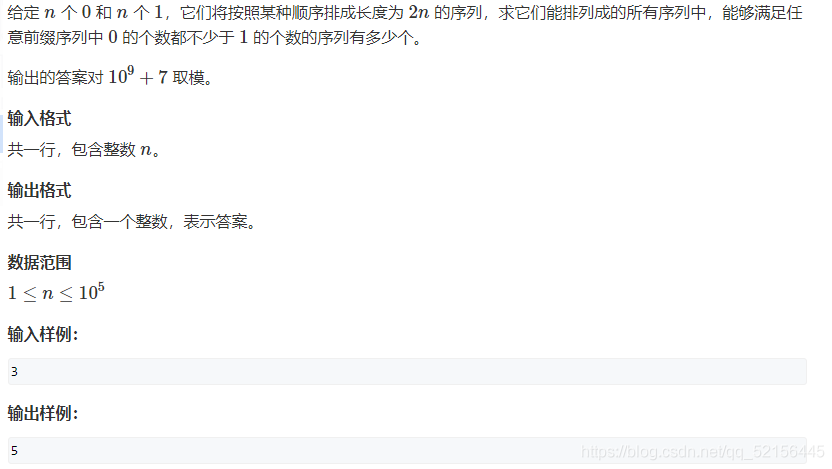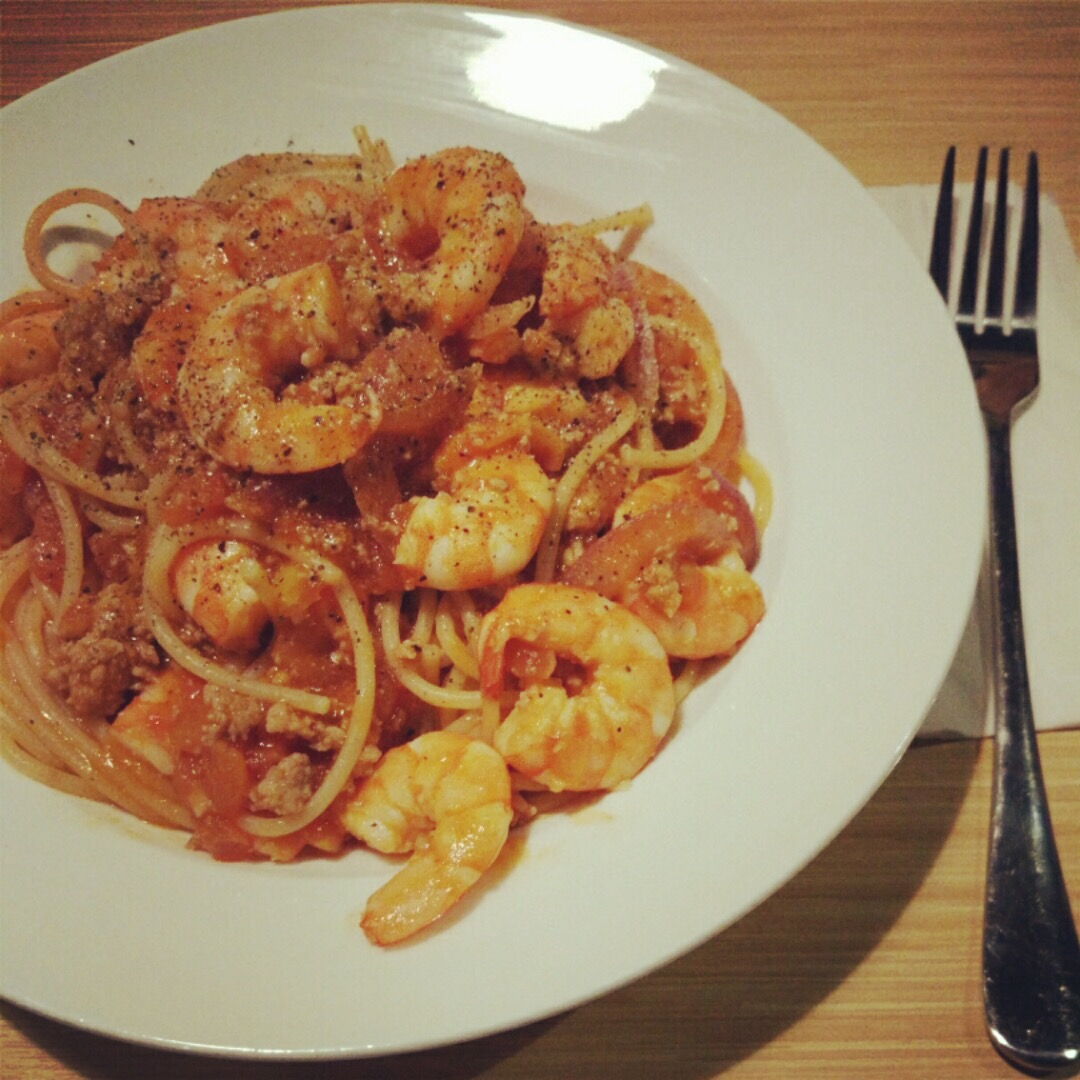# 母函数与排列组合

+关注继续查看

（1+x）*（1+x+x2）*（1+x3）=1+2x+2x2+2x3+2x4+2x5+x6

这个多项式和上面的有一些区别了，它的幂级数超过1了。如果要从（1+x）、（1+x+x2）和（1+x3）中得到x的2次方的话，有两种选择：从（1+x）和（1+x+x2）中分别选择一个x或者从（1+x+x2）中选择x2；如果要得到x的6次方的话，只有1种选择，就是从（1+x）中选择x、（1+x+x2）中选择x2、（1+x3）中选择x3。也就是说乘积结果的每一项anxn的前面的系数an表示了从（1+x）、（1+x+x2）和（1+x3）中得到xn的组合数。

其实上面的例子就利用了母函数的思想，下面来具体讨论一下母函数。

下面这个对于母函数的描述摘自维基百科：

也就是说母函数是针对某个序列的，它的外在表现形式是一种形式幂级数。比如说有这样一个序列a0，a1，......an，构造一个函数

f(x)=a0+a1x+a2x2+......+anxn

则f(x)是序列a0，a1，......an的母函数。比如说最常见的(1+x)n，它是序列C(n,0)，C(n,1)，C(n,2)...C(n,n)的母函数。

母函数包括几种，其中最常见的是普通型母函数和指数型母函数。普通型母函数是形如　f(x)=a0+a1x+a2x2+......+anxn的函数，而指数型母函数是形如G(x) = a0 + a1*(x)/1! + a2*(x2)/ 2! + a3*(x3)/3! + …… an*(xn)/k!的函数。

利用母函数的思想可以解决很多组合问题，下面举例说明：

1.口袋中有白球2个，红球3个，黄球1个，从袋中摸出3个球有几种取法？

和上面描述的例子类似，我们可以用次数代表球的个数，多项式的每一项前面的系数代表取法的种树。

可以很容易地写出下面这个式子：

（1+x+x2）（1+x+x2+x3）（1+x）

（1+x+x2）表示有白球2个，1表示白球不取，x代表取1个白球，x2代表取2个白球，即用x的次数表示取球的个数，后面的也是类似。那么这个多项式的乘积就把所有的情况都表示出来了，对于最终乘积的每一项anxn，表示取n个球有an种取法。

2.有若干个1克，2克，5克的砝码，要称出20克的重量，有多少种称法？

这里不限制砝码的个数，无所谓，照样写出母函数：

（1+x+x2+x3+......xk+....）（1+x2+x4+x6......+x2n+......）（1+x5+x10+......x5m+......）

因为要称出20克，所以只需要找找到结果中次数为20 的那一项就可以得到结果。

3.同样对于正数划分也可以解决，比如有整数20，划分成1，2，5，有多少种划分方法？

解法和2的类似。

还有一类题目和这类似，有n个球放到m个盒子中，有多少种不同的放法？

（1+x+x2+x3+...xk+...）（1+x+x2+x3+...xk+...）（1+x+x2+x3+...xk+...）总共有m项，然后找出乘积中次数为n的那一项系数。

1.口袋中有白球2个，红球3个，黄球1个，任取3个作为一个排列，总共有多少种排列？

类似地用指数型母函数解决

用（1+x/1!+x2/2!）表示取白球0个，1个或者2个

那么（1+x/1!+x2/2!）（1+x/1!+x2/2!+x3/3!）（1+x/1!）来表示所有的排列结果。

=1+3x+4x2+19x3/6+19x4/12+6x5/12+x6/12

=1+3*(x/1!)+8*(x2/2!)+19*(x3/3!)+38*(x4/4!)+60*(x5/5!)+60*(x6/6!)

找到次数为3的那一项，系数为19，那么总共有19种排列。

2.用1，2，3，4能够组成多少个5位数，要求1出现2次或者3次，2出现0次或者1次，3没有限制，4只出现偶数次。

（x2/2!+x3/3!）（1+x）（1+x/1!+x2/2!+x3/3!+.....xk/k!+....）（1+x2/2!+x4/4!+......+x2n/(2n)!+......）

每个式子的含义就不多解释了，读者应该能看懂它的含义。最终的结果就是x5/5!这一项的系数。

用代码去实现母函数的计算过程很简单，它是模拟我们人工计算多项式乘积的过程，比如有多项式H1*H2*H3......

我们先计算H1和H2的乘积，得到结果H'，再用H'和H3相乘......依次类推下去，直到得到最终的结果。

http://acm.hdu.edu.cn/showproblem.php?pid=1085

#include <iostream>
using namespace std;

int main(int argc, char *argv[])
{
int num;
int cent={1,2,5};          //次数增长步长
while(scanf("%d %d %d",&num,&num,&num) != EOF)
{
if(num==0&&num==0&&num==0)
break;
int i,j,k;
int c,tempC;     //因为三种硬币最多1000枚，1*1000+2*1000+5*1000=8000，那么多项式乘积的最高次数为8000
//c保存累计相乘各项的系数，tempC保存c和当前项相乘的系数
//c[i] 表示次数为i的那一项的系数

int max=num+2*num+5*num;   //求出该组输入条件下的最高次数

for(i=0;i<=8001;i++)
{
c[i]=0;
tempC[i]=0;
}

//母函数为(1+x+x^2+...x^num)(1+x^2+x^4+....x^2*num)(1+x^5+x^10+...+x^5*num)
for(i=0;i<=cent*num;i+=cent)   //第一个多项式的系数初始化
{
c[i]=1;
}

for(i=1;i<3;i++)    //i表示总共有多少个多项式
{
for(j=0;j<=max;j++)    //累计相乘的x^j的系数
{
for(k=0;k+j<=max && k<=cent[i]*num[i];k+=cent[i])   //当前项x^k的系数
{
tempC[k+j]+=c[j];   //x^j * x^k=x^(j+k)
}
}

for(j=0;j<=max;j++)    //将临时数组清零
{
c[j]=tempC[j];
tempC[j]=0;
}
}

for(i=0;i<=max;i++)
{
if(c[i]==0)
break;
}
printf("%d\n",i);
}
return 0;
}0 0【组合数学】排列组合 ( 排列组合示例 )
【组合数学】排列组合 ( 排列组合示例 )
0 0668 01110 0amb解决排列组合问题
1321 01329 0748 0576 0437 0
usher.yue